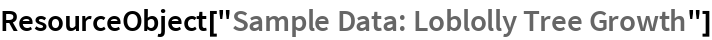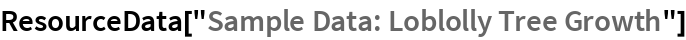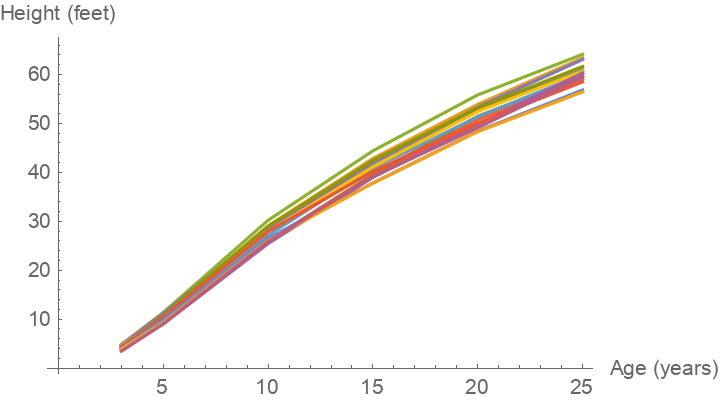# Sample Data: Loblolly Tree Growth

Loblolly pine tree growth measurement

Originator: F. H. Kung

Time series data on the increase of tree height measured for each of 14 trees from different seed sources.

## Examples

### Basic Examples

Retrieve the ResourceObject:

 In:=Out=View the data:

 In:=Out=### Visualization

Plot the height of the trees over time:

 In:=Out=Wolfram Research, "Sample Data: Loblolly Tree Growth" from the Wolfram Data Repository (2016) https://doi.org/10.24097/wolfram.67358.data